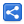# Final vs Effectively Final

[Last Updated: Feb 10, 2016]

A variable or parameter whose value never changes after it is initialized, is effectively final.

A variable or parameter declared with the final keyword is final.

Starting in Java SE 8, a local class or lambda expression can access local variables and parameters of the enclosing block that are final (old feature) or effectively final (new feature).

### Examples of effectively final variables:

In following example, since value of x never changes after its initialization, it's effectively final and can be used in local class or lambda expressions:

``` String x = "some str";
new Runnable(){
@Override
public void run() {
System.out.println(x);
}
};```

Lambda expression example of effectively final:

```    String x = "some str";
Runnable r = ()-> System.out.println(x+" appended");```

```public void doSomething(String x) {
Runnable r = () -> System.out.println(x + " appended");
}```

Not 'effectively final' example:

```    String x = "some str";
x = "assinged again";
Runnable r = ()-> System.out.println(x+" appended");
```

Since the value of x changed in the second line, we have compile time error.

Output:

Compiler error:
Error:(10, 47) java: local variables referenced from a lambda expression must be final or effectively final

Following will also be an error, even value of x changes after lambda expression.

```    String x = "some str";
Runnable r = () -> System.out.println(x + " appended");
x = "assinged again";```

Output:

Compiler error:
Error:(9, 47) java: local variables referenced from a lambda expression must be final or effectively final

 Share